# Gp - 80

Sum of the first four members of a geometric progression is 80. Determine they if we know that the fourth member is nine times greater than the second.

Result

a1 =  2
a2 =  6
a3 =  18
a4 =  54
b1 =  -4
b2 =  12
b3 =  -36
b4 =  108

#### Solution:Leave us a comment of example and its solution (i.e. if it is still somewhat unclear...):

Showing 0 comments:Be the first to comment!## Next similar examples:

1. Eight palm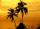There grows 8 palms by the sea. At the first sitting one parrot, on second two, on third sits four parrots on each other twice the previous parrots sitting on a previous palm. How many parrots sitting on eighth palm?
2. GP - 8 itemsDetermine the first eight members of a geometric progression if a9=512, q=2
3. Five membersWrite first 5 members geometric sequence and determine whether it is increasing or decreasing: a1 = 3 q = -2
4. Geometric sequence 4It is given geometric sequence a3 = 7 and a12 = 3. Calculate s23 (= sum of the first 23 members of the sequence).
5. Six termsFind the first six terms of the sequence a1 = -3, an = 2 * an-1
6. Computer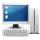The computer was purchased 10000,-. Each year, the price of a computer depreciates always the same percentage of the previous year. After four years, the value of the computer is reduced to 1300,- How many percent was depreciated price of the computer each
7. Quotient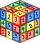Determine the quotient and the second member of the geometric progression where a3=10, a1+a2=-1,6 a1-a2=2,4.
8. Tenth memberCalculate the tenth member of geometric sequence when given: a1=1/2 and q=2
9. Geometric progression 2There is geometric sequence with a1=5.7 and quotient q=-2.5. Calculate a17.
10. Geometric progression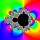Fill 4 numbers between 4 and -12500 to form geometric progression.
11. Piano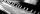If Suzan practicing 10 minutes at Monday; every other day she wants to practice 2 times as much as the previous day, how many hours and minutes will have to practice on Friday?
12. Geometric progression 48,4√2,4,2√2
13. Ray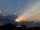Light ray loses 1/19 of brightness passing through glass plate. What is the brightness of the ray after passing through 7 identical plates?
14. Theorem proveWe want to prove the sentense: If the natural number n is divisible by six, then n is divisible by three. From what assumption we started?
15. PowerNumber ?. Find the value of x.
16. CalculationHow much is sum of square root of six and the square root of 225?
17. EquationHow many real roots has equation ? ?